# Physical Chemistry : Molecules

## Example Questions

### Example Question #1 : Covalent Bonding

Which statement best characterizes a covalent bond?

Cations and anions

Electrical conduction

Electron sea

Crystal structure

Sharing electrons between atoms

Sharing electrons between atoms

Explanation:

To achieve an octet of valence electrons, atoms can share electrons so that all atoms participating in the bond will have full valence shells. Covalent bonds, by definition, result from the sharing of one or more pairs of valence electrons.

### Example Question #1 : Covalent Bonding

How many single covalent bonds would the element sulfur be expected to form in order to obey the octet rule?Explanation:

The key to this problem is that electrons in covalent bonds are shared and therefore "belong" to both of the bonded atoms. Sulfur is a nonmetal in group 6A , and therefore has 6 valence electrons. In order to obey the octet rule, it needs to gain 2 electrons . It can do this by forming 2 single covalent bonds.

### Example Question #21 : Nuclear, Quantum, And Molecular Chemistry

How many single covalent bonds would the element selenium be expected to form in order to obey the octet rule?0Explanation:

The key to this problem is that electrons in covalent bonds are shared and therefore "belong" to both of the bonded atoms. Selenium is a nonmetal in group 6A , and therefore has 6 valence electrons. In order to obey the octet rule, it needs to gain 2 electrons. It can do this by forming 2 single covalent bonds.

### Example Question #2 : Covalent Bonding

Which of the following constitutes a covalent bond?

All of these

Electron pair shared between two neighboring atoms

Atoms of similar electronegativities

Spin pairing of electrons

Constructive interference between atomic orbitals

All of these

Explanation:

A chemical bond is considered covalent if there is sharing of one or more pairs of electrons between atoms. As opposed to a covalent bond, an ionic bond can involve the transfer of electrons from one atom to another resulting in a high charge differential between two atoms in order for them to acquire a full octet.

As described by the Pauli Exclusion Principle, every pair of electrons must consist of spin-up paired with spin-down. It states that no more than two electrons may occupy an orbital, and in full electron orbitals, the spin of one must cancel the spin of the other so their spins will have a zero net spin/angular momentum.

Chemical bonds are made up of orbitals, which are simply waves that have wave functions. Wave functions tell us the likelihood that an electron can be found within an orbital. Constructive interference of two wave functions/orbitals occur when the two waves are in phase and result in a new wave function, or in other words a chemical bond.

### Example Question #1 : Molecules

Which of the following molecules does not contain polar bonds?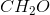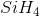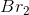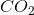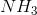Explanation:

Polar bonds form between all atoms of different electronegativity. Bromine is a diatomic molecule and thus both atoms of bromine have the same electronegativity. This bond between bromine is perfectly nonpolar, meaning that both atoms share the electron density equally.

### Example Question #1 : Molecules

Which of the following molecules has the smallest bond angle?

Ammonia

Methane

Water

Carbon dioxide

Water

Explanation:

One of the biggest factors that can affect the bond angles in a molecule is the presence of lone pairs. A general rule is that each lone pair will decrease the predicted bond angle by about two degrees. Methane, for example, has no lone pairs on the central carbon, so its bond angles are 109.5 degrees. Ammonia, however, has one lone pair, which makes its angles closer to 107.5 degrees. Finally, water has 2 lone pairs, giving it a bond angle of about 105 degrees.

### Example Question #1 : Molecules

Which molecule exhibits trigonal pyramidal geometry?

Methane

Boron trifluoride

Carbon dioxide

Ammonia

Ammonia

Explanation:

A trigonal pyramidal geometry is witnessed when molecules have a central atom bonding to three additional atoms and one lone pair. The nitrogen atom in ammonia has three hydrogens attached, as well as a lone pair, in order to satisfy its octet. This gives ammonia a trigonal pyramidal geometry.

Methane has a tetrahedral geometry, carbon dioxide is linear, and boron trifluoride is trigonal planar.

### Example Question #1 : Molecular Geometry And Vsepr

A metal with sp3d2 hybridized orbitals contains eight valence electrons. If four monodentate ligands are bound to this metal, what is the resulting molecular geometry?

tetrahedral

square pyramidal

square planar

seesaw

T-shaped